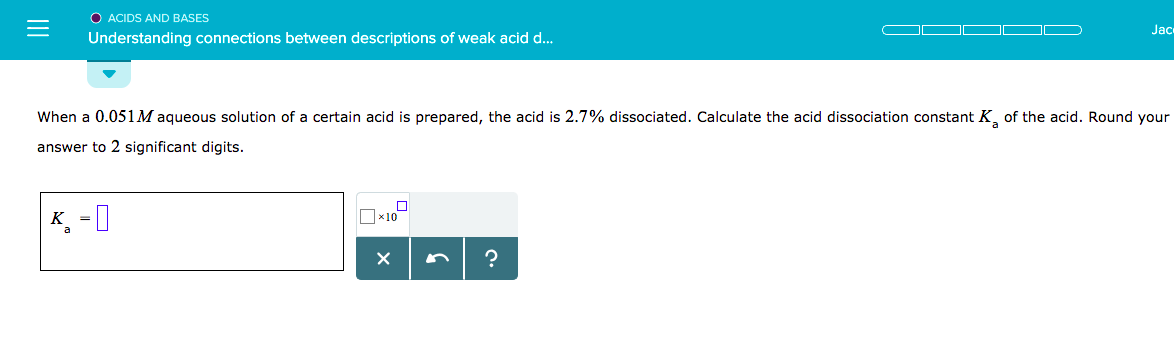O ACIDS AND BASESUnderstanding connections between descriptions of weak acid d...Jacwhen a 0.051 M aqueous solution of a certain acid is prepared, the acid is 2.7% dissociated. Calculate theanswer to 2 significant digits.acid dissociation constant K, ofthe acid. Round your

Question

Acids & Bases: Understanding Connections Between Descriptions of Weak Acid Dissociation

When a 0.051M aqueous solution of a certain acid is prepared, the acid is 2.7% dissociated. Calculate the acid dissociation constant Ka of the acid. Round your answer to 2 significant digits.

*PHOTO INCLUDED BELOWhelp_outlineImage TranscriptioncloseO ACIDS AND BASES Understanding connections between descriptions of weak acid d... Jac when a 0.051 M aqueous solution of a certain acid is prepared, the acid is 2.7% dissociated. Calculate the answer to 2 significant digits. acid dissociation constant K, of the acid. Round your fullscreen
Step 1

The percent ionization constant of a weak acid is given as 2.7%. The initial molarity of the acid is 0.051 M.

Let the ionization of the acid be denoted as

The acid ionization constant is given as

Step 2

Since the percent ionization and the initial concentrations are given, the concentration of proton, H+ at equilibrium can be easily calculated.

Due to the 1:1 nature of ionization, [A-] = 0.001377 M.

Since the acid HA has undergo dissociation, the equilibrium concentration of HA can be determined as

Step 3

Plug in the values of [H+], [A-] and [HA] at equilibrium ...

Want to see the full answer?

See Solution

Want to see this answer and more?

Our solutions are written by experts, many with advanced degrees, and available 24/7

See Solution
Tagged in

Chemistry